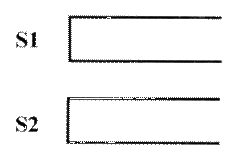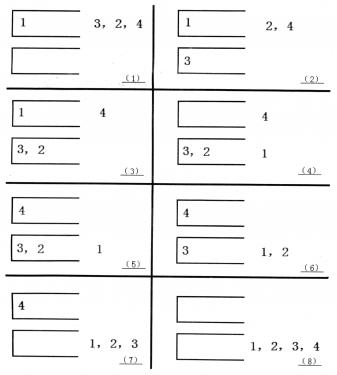# P1155 双栈排序

• 1.7K通过
• 4.6K提交
• 题目提供者 CCF_NOI
• 评测方式 云端评测
• 标签 图论 NOIp提高组 2008
• 难度 提高+/省选-
• 时空限制 1000ms / 128MB
• 提示：收藏到任务计划后，可在首页查看。

## 题目描述

Tom最近在研究一个有趣的排序问题。如图所示，通过$2$个栈$S_1$和$S_2$，Tom希望借助以下$4$种操作实现将输入序列升序排序。操作$a$

如果输入序列不为空，将第一个元素压入栈$S_1$

操作$b$

如果栈$S_1$不为空，将$S_1$栈顶元素弹出至输出序列

操作$c$

如果输入序列不为空，将第一个元素压入栈$S_2$

操作$d$

如果栈$S_2$不为空，将$S_2$栈顶元素弹出至输出序列

如果一个$1-n$的排列P可以通过一系列操作使得输出序列为$1,2,…,(n-1),n$，Tom就称$P$是一个“可双栈排序排列”。例如$(1,3,2,4)$就是一个“可双栈排序序列”，而$(2,3,4,1)$不是。下图描述了一个将$(1,3,2,4)$排序的操作序列：$<a,c,c,b,a,d,d,b>$当然，这样的操作序列有可能有几个，对于上例$(1,3,2,4)$，$<a,c,c,b,a,d,d,b>$是另外一个可行的操作序列。Tom希望知道其中字典序最小的操作序列是什么。

## 输入输出格式

输入格式：

第一行是一个整数$n$。

第二行有$n$个用空格隔开的正整数，构成一个$1-n$的排列。

输出格式：

共一行，如果输入的排列不是“可双栈排序排列”，输出数字$0$；否则输出字典序最小的操作序列，每两个操作之间用空格隔开，行尾没有空格。

## 输入输出样例

输入样例#1： 复制
4
1 3 2 4
输出样例#1： 复制
a b a a b b a b
输入样例#2： 复制
4
2 3 4 1
输出样例#2： 复制
0
输入样例#3： 复制
3
2 3 1
输出样例#3： 复制
a c a b b d

## 说明

$30\%$的数据满足： $n \le 10$

$50\%$的数据满足：$n \le 50$

$100\%$的数据满足： $n \le 1000$

提示
标程仅供做题后或实在无思路时参考。
请自觉、自律地使用该功能并请对自己的学习负责。
如果发现恶意抄袭标程，将按照I类违反进行处理。